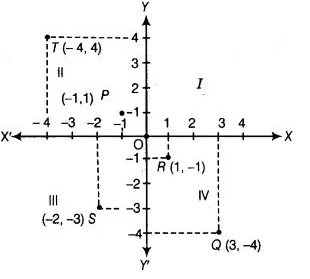# If P(-l, 1), Q(3, -4), R(1, -1), S(-2, -3) and T(-4, 4)`
Question:

If P(-l, 1), Q(3, -4), R(1, -1), S(-2, -3) and T(-4, 4) are plotted on the graph paper, then the point(s) in the fourth quadrant is/are

(a) P and

(b) Q and R

(c) Only S

(d) P and R

Solution:

(b) In point P (-1, 1), x-coordinate is -1 unit and y-coordinate is 1 unit, so it lies in llnd quadrant.

Similarly, we can plot all the points Q (3, -4), R (1, -1), S (-2, -3) and T (-4, 4),

It is clear from the graph that points R and Q lie in fourth quadrant.